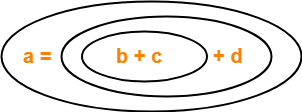# Three Address Code | Examples

 Three Address Code is a form of an intermediate code.

The characteristics of Three Address instructions are-

• They are generated by the compiler for implementing Code Optimization.
• They use maximum three addresses to represent any statement.
• They are implemented as a record with the address fields.

## General Form-

In general, Three Address instructions are represented as-

 a = b op c

Here,

• a, b and c are the operands.
• Operands may be constants, names, or compiler generated temporaries.
• op represents the operator.

### Examples-

Examples of Three Address instructions are-

• a = b + c
• c = a x b

## Common Three Address Instruction Forms-

The common forms of Three Address instructions are-

### 1. Assignment Statement-

 x = y op z and x = op y

Here,

• x, y and z are the operands.
• op represents the operator.

It assigns the result obtained after solving the right side expression of the assignment operator to the left side operand.

### 2. Copy Statement-

 x = y

Here,

• x and y are the operands.
• = is an assignment operator.

It copies and assigns the value of operand y to operand x.

### 3. Conditional Jump-

 If x relop y goto X

Here,

• x & y are the operands.
• X is the tag or label of the target statement.
• relop is a relational operator.

If the condition “x relop y” gets satisfied, then-

• The control is sent directly to the location specified by label X.
• All the statements in between are skipped.

If the condition “x relop y” fails, then-

• The control is not sent to the location specified by label X.
• The next statement appearing in the usual sequence is executed.

### 4. Unconditional Jump-

 goto X

Here, X is the tag or label of the target statement.

On executing the statement,

• The control is sent directly to the location specified by label X.
• All the statements in between are skipped.

### 5. Procedure Call-

 param x call p return y

Here, p is a function which takes x as a parameter and returns y.

## PRACTICE PROBLEMS BASED ON THREE ADDRESS CODE-

To solve the problems, Learn about the Precedence Relations and Associativity of Operators.

## Problem-01:

Write Three Address Code for the following expression-

a = b + c + d

## Solution-

The given expression will be solved as-Three Address Code for the given expression is-

(1) T1 = b + c

(2) T2 = T1 + d

(3) a = T2

## Problem-02:

Write Three Address Code for the following expression-

-(a x b) + (c + d) – (a + b + c + d)

## Solution-

Three Address Code for the given expression is-

(1) T1 = a x b

(2) T2 = uminus T1

(3) T3 = c + d

(4) T4 = T2 + T3

(5) T5 = a + b

(6) T6 = T3 + T5

(7) T7 = T4 – T6

## Problem-03:

Write Three Address Code for the following expression-

If A < B then 1 else 0

## Solution-

Three Address Code for the given expression is-

(1) If (A < B) goto (4)

(2) T1 = 0

(3) goto (5)

(4) T1 = 1

(5)

## Problem-04:

Write Three Address Code for the following expression-

If A < B and C < D then t = 1 else t = 0

### Solution-

Three Address Code for the given expression is-

(1) If (A < B) goto (3)

(2) goto (4)

(3) If (C < D) goto (6)

(4) t = 0

(5) goto (7)

(6) t = 1

(7)

Watch this Video LectureNext Article- Quadruples, Triples & Indirect Triples

Get more notes and other study material of Compiler Design.

Watch video lectures by visiting our YouTube channel LearnVidFun.

SummaryArticle Name